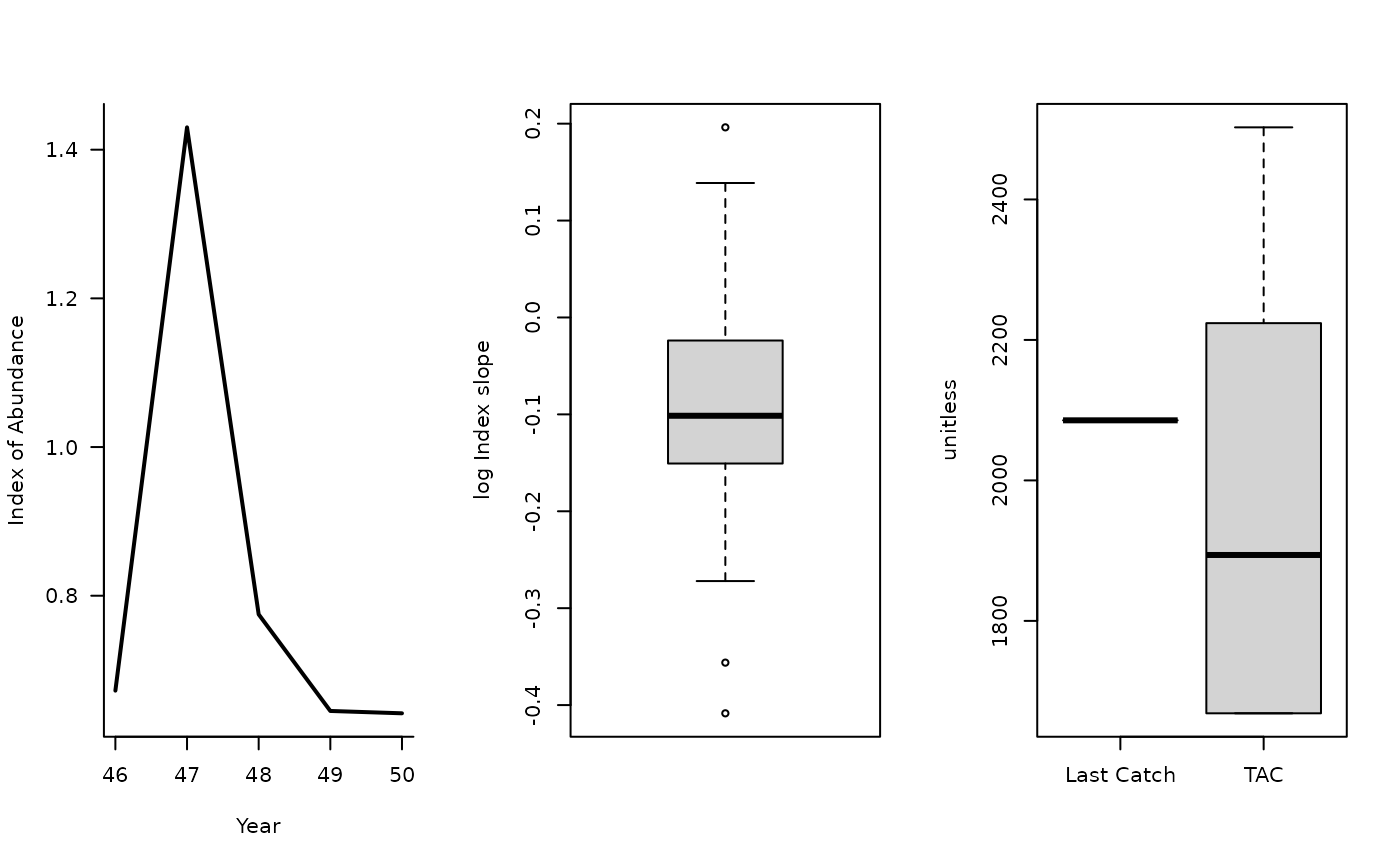An MP similar to SBT1 that modifies a time-series of catch recommendations and aims for a stable catch rates.

GB_slope(x, Data, reps = 100, plot = FALSE, yrsmth = 5, lambda = 1)

## Arguments

x

A position in the data object

Data

A data object

reps

The number of stochastic samples of the MP recommendation(s)

plot

Logical. Show the plot?

yrsmth

Number of years for evaluating slope in relative abundance index

lambda

A gain parameter

## Value

An object of class Rec-class with the TAC slot populated with a numeric vector of length reps

## Details

The TAC is calculated as: $$\textrm{TAC}_y= C_{y-1} \left(1+\lambda I\right)$$ where $$C_{y-1}$$ is catch from the previous year, $$\lambda$$ is a gain parameter, and $$I$$ is the slope of the linear regression of log Index (Data@Ind) over the last yrsmth years.

The TAC is subject to the following conditions:

1. if next TAC > 1.2 last catch, then TAC = 1.2 last catch

2. if next TAC < 0.8 last catch, then TAC = 0.8 last catch

Note that this is my interpretation of their approach and is now stochastic. Currently it is generalized and is not 'tuned' to more detailed assessment data which might explain why in some cases it leads to stock declines.

## Required Data

See Data-class for information on the Data object

GB_slope: Cat, Ind, Year

## Rendered Equations

See Online Documentation for correctly rendered equations

Other Index methods: GB_target(), Gcontrol(), ICI(), Iratio(), Islope1(), Itarget1_MPA(), Itarget1(), ItargetE1()

T. Carruthers

## Examples

GB_slope(1, MSEtool::SimulatedData, plot=TRUE)#> TAC (median)
#>     1775.624